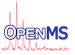OpenMS  2.8.0
CubicSpline2d Class Reference

cubic spline interpolation as described in R.L. Burden, J.D. Faires, Numerical Analysis, 4th ed. PWS-Kent, 1989, ISBN 0-53491-585-X, pp. 126-131. More...

`#include <OpenMS/MATH/MISC/CubicSpline2d.h>`

Collaboration diagram for CubicSpline2d:
[legend]

## Public Member Functions

CubicSpline2d (const std::vector< double > &x, const std::vector< double > &y)
constructor of spline interpolation More...

CubicSpline2d (const std::map< double, double > &m)
constructor of spline interpolation More...

double eval (double x) const
evaluates the spline at position x More...

double derivative (double x) const
evaluates first derivative of spline at position x More...

double derivatives (double x, unsigned order) const
evaluates derivative of spline at position x More...

## Private Member Functions

void init_ (const std::vector< double > &x, const std::vector< double > &y)
initialize the spline More...

## Private Attributes

std::vector< double > a_
constant spline coefficients More...

std::vector< double > b_
linear spline coefficients More...

std::vector< double > c_

std::vector< double > d_
cubic spline coefficients More...

std::vector< double > x_
knots More...

## Detailed Description

cubic spline interpolation as described in R.L. Burden, J.D. Faires, Numerical Analysis, 4th ed. PWS-Kent, 1989, ISBN 0-53491-585-X, pp. 126-131.

Construction of the spline takes by far the most time. Evaluating it is rather fast (one evaluation is about 50x faster than construction – depending on number of points etc.).

## ◆ CubicSpline2d() [1/2]

 CubicSpline2d ( const std::vector< double > & x, const std::vector< double > & y )

constructor of spline interpolation

The coordinates must match by index. Both vectors must be the same size and sorted in x. Sortedness in x is required for

SplinePackage.
Parameters
 x x-coordinates of input data points (knots) y y-coordinates of input data points

## ◆ CubicSpline2d() [2/2]

 CubicSpline2d ( const std::map< double, double > & m )

constructor of spline interpolation

Parameters
 m (x,y) coordinates of input data points

## ◆ derivative()

 double derivative ( double x ) const

evaluates first derivative of spline at position x

Parameters
 x x-position

## ◆ derivatives()

 double derivatives ( double x, unsigned order ) const

evaluates derivative of spline at position x

Parameters
 x x-position order order of the derivative Only order 1 or 2 make sense for cubic splines.

## ◆ eval()

 double eval ( double x ) const

evaluates the spline at position x

Parameters
 x x-position

## ◆ init_()

 void init_ ( const std::vector< double > & x, const std::vector< double > & y )
private

initialize the spline

Parameters
 x x-coordinates of input data points (knots) y y-coordinates of input data points

## ◆ a_

 std::vector a_
private

constant spline coefficients

## ◆ b_

 std::vector b_
private

linear spline coefficients

## ◆ c_

 std::vector c_
private

## ◆ d_

 std::vector d_
private

cubic spline coefficients

## ◆ x_

 std::vector x_
private

knots